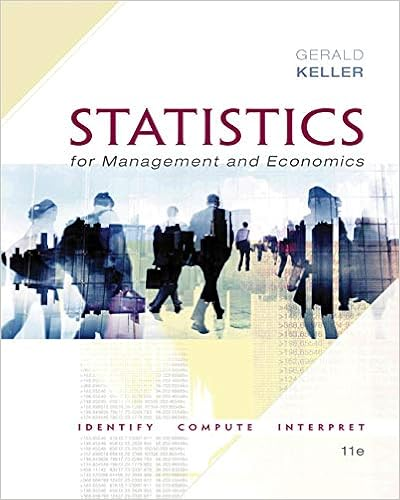# Only drawback data table can be invoked only once in

• Notes
• 13
• 80% (5) 4 out of 5 people found this document helpful

This preview shows page 12 - 13 out of 13 pages.

##### We have textbook solutions for you!
The document you are viewing contains questions related to this textbook.The document you are viewing contains questions related to this textbook.
Chapter 22 / Exercise 22.8
Statistics for Management and Economics + XLSTAT Bind-in
KellerExpert Verified
Only drawback: Data Table can be invoked only once in each execution. In other words, wecannot have two layers of Data Tables.See attachedDataTable.pdffor detail.How To Speed Up Excel Execution:seeIndicators and Logical Operations:Logical functions in Excel, hit F1 for help in Excel:Function DescriptionAND Returns TRUE if all of its arguments are TRUEFALSE Returns the logical value FALSEIF Specifies a logical test to performNOT Reverses the logic of its argumentOR Returns TRUE if any argument is TRUETRUE Returns the logical value TRUEHowever, using the notion of indicator, we can greatly simply our work. We summarizelogical operations as follows.IndicatorLetIAbe theindicatorof event (statement)A(e.g.,D > S), i.e, the logical value ofthe statement:IA= 1ifAis true; 0 otherwise. In Exel:IF(A,1,0), or simply1*(A).CausalityABdenotesAis a subset ofB, i.e.,AimpliesB.ORABbe the union ofAandB. In Excel:OR(A,B), or(A+B)if we interpret positivenumbers as TRUE.ANDABthe intersection ofAandB. In Excel:AND(A,B), or(A*B).NOTAc, i.e., notA. In Excel:NOT(A), or1-A.Moreover, letA\BbeABc, i.e.,Abut notB,A×Bthe Cartesian product ofAandB.LetXdenote the entire sample space. We have the following useful properties for generalindicator functions:I= 0IX= 1ABIAIBIAB=IA·IB= min(IA, IB)IAB=IA+IB-IA·IB= max(IA, IB)IA\B=IA-IA·IBIA×B=IA·IB.12
##### We have textbook solutions for you!
The document you are viewing contains questions related to this textbook.The document you are viewing contains questions related to this textbook.
Chapter 22 / Exercise 22.8
Statistics for Management and Economics + XLSTAT Bind-in
KellerExpert Verified
Random sample size:In Excel, the sample size is usually given, e.g.,N= 1000. So after generating the first ob-servation, you can simply drag down to get the whole sample. However, when the samplesize is random, e.g., in the insurance model, you need the following trick. The key is useindicator smartly.Consider the grades of simulation class. The gradeXiof each studentiis continuous uni-formly distributed over[90,100], i.e.,XU[0,10]. We are interested in thetotal team gradesY, the sum of all members’ grades of a team. It is known that the team sizeNfollows dis-crete uniform distribution over{1,2,3,4}. Therefore, for a randomly picked team, its totalgrades isY=Ni=1Xi. What is the distribution ofY? Assume sample sizen= 50(i.e.,simulate 50 teams).
•••